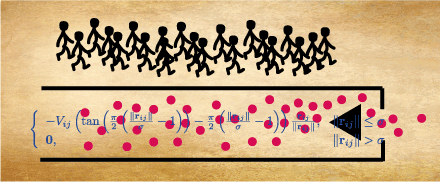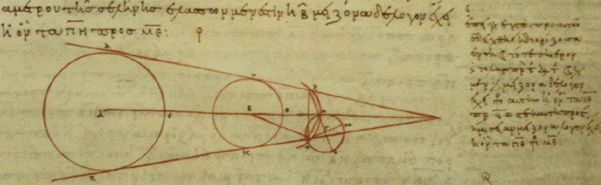researcha brief CVresearchteachinglinksacknowledgements
 Industrial Mathematics Connecting research mathematics with industrial problem solving is one of the most exciting and rewarding activities I have taken part in. Discovering the surprisingly complex and often difficult mathematical issues at play in very down-to-earth settings is a source of wonder, inspiration and hard work. Many different fields of mathematics may come into play. Working with others is highly recommended.Crowd Dynamics The study of crowd motions is important because increasing populations concentrations in the built environment require an understanding of the complex dynamics of many agents moving and simultaneously interacting with each other and with physical boundaries, walls, and obstacles. A mix of dynamical systems theory, experiments and data analysis from simulations is able to make progress in this area where lack of first-principles presents a special challenge.Dynamical Systems, Classical Mechanics. The science of Dynamical Systems is the study of systems that evolve according to some underlying principle. A large number of mathematical techniques are involved; often ordinary or partial differential equations (when the system changes smoothly). Classical Mechanics is the study of mechanical systems that are governed by Newton's, Lagrange's, Hamilton's, or Einstein's equations of motion (as opposed to, e.g., quantum mechanical equations of motion).Finite Metric Spaces, Global Analysis, Differential Geometry. Among the mathematical tools used in the study of dynamical systems are the subjects of Differential Geometry, and, more generally, Global Analysis. They are also beautiful and profound mathematical disciplines in their own right. Recently I have explored, with S. Markvorsen and others, the connecetions between the linear algebra of metric matrices (the metric information in a finite metric space) and the geometry of the (potentially) imbedding space. Which geometric properties of the space reveal themselves as matrix properties of generic metric matrices? Only one thing is clear: the final word. has not yet been spoken.